시간 제한메모리 제한제출정답맞은 사람정답 비율
15 초 (추가 시간 없음) 1024 MB (추가 메모리 없음)933100.000%

## 문제

We have a matrix of square cells with R rows and C columns. We need to draw a diagonal in each cell. Exactly one of two possible diagonals must be drawn in each cell: the forward slash diagonal, which connects the bottom-left and the top-right corners of the cell, or the backslash diagonal, which connects the top-left and the bottom-right corners of the cell.

For each row and column, we want to draw a specific number of diagonals of each type. Also, after all the diagonals are drawn, the matrix should be square free. That is, there should be no squares formed using the diagonals we added.

For example, suppose we have a matrix with 4 rows and 4 columns. The number next to each row is the exact number of forward slash diagonals there must be in that row. The number below each column is the exact number of forward slash diagonals there must be in that column.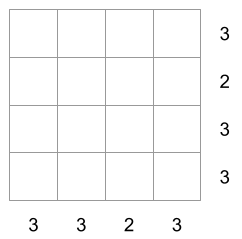There are multiple ways to fill the matrix respecting those per-row and per-column amounts. Below we depict three possibilities: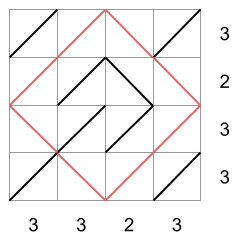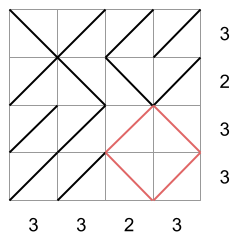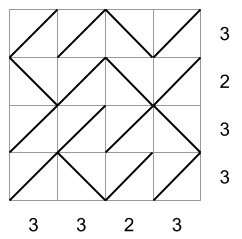The first two matrices are not square free, while the third matrix is. In the first matrix, there is a square of side-length 2 diagonals with its vertices in the middle of each side of the matrix. In the second matrix, there is a square of side-length 1 diagonal drawn in the bottom-right corner. In the third matrix, there is no square. The third matrix would then be a valid drawing according to all the rules.

Given the size of the matrix and the exact number of forward slash diagonals that must be drawn in each row and column, produce any square free matrix that satisfies the row and column constraints, or say that one does not exist.

## 입력

The first line of the input gives the number of test cases, T. T test cases follow. Each test case consists of exactly three lines. The first line of a test case contains R and C, the number of rows and columns of the matrix. The second line of a test case contains R integers S1, S2, …, SR. Si is the exact number of forward slash diagonals that must be drawn in the i⁠-⁠th row from the top. The third line of a test case contains C integers D1, D2, …, DC. Di is the exact number of forward slash diagonals that must be drawn in the i⁠-⁠th column from the left.

## 출력

For each test case, output one line containing Case #x: y, where x is the test case number (starting from 1) and y is IMPOSSIBLE if there is no filled matrix that follows all rules and POSSIBLE otherwise. If you output POSSIBLE, output R more lines with C characters each. The j-⁠th character of the i-⁠th of these lines must be / if the diagonal drawn in the i-⁠th row from the top and j-⁠th column from the left in your proposed matrix is a forward slash diagonal, and \ otherwise. Your proposed matrix must be valid according to all rules.

## 제한

• 1 ≤ T ≤ 100.
• 0 ≤ Si ≤ C, for all i.
• 0 ≤ Di ≤ R, for all i.

• 2 ≤ R ≤ 6.
• 2 ≤ C ≤ 6.

• 2 ≤ R ≤ 20.
• 2 ≤ C ≤ 20.

## 예제 입력 1

4
4 4
3 2 3 3
3 3 2 3
2 3
1 1
1 1 1
2 3
1 2
1 1 1
3 3
2 0 2
2 0 2


## 예제 출력 1

Case #1: POSSIBLE
//\/
\/\/
///\
/\//
Case #2: IMPOSSIBLE
Case #3: POSSIBLE
\\/
//\
Case #4: POSSIBLE
/\/
\\\
/\/


## 힌트

Sample Case #1 is the one explained above.

In Sample Case #2, there must be a total of 2 forward slash diagonals according to the sum of the row totals, but a total of 3 according to the sum of the column totals. It is therefore impossible to follow all rules.

In Sample Case #3 the only matrices that follow the row and column totals are the following: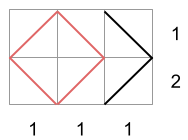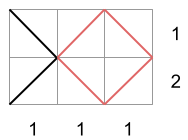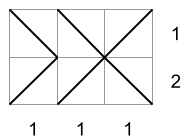Since the first two contain a square, the third one is the only valid output for this case.

In Sample Case #4 there is only one way to fill the matrix that follows the row and column totals, shown in the picture below. Note that it produces a single rectangle, shown in blue in the picture. But, since that rectangle is not a square, the matrix is square free.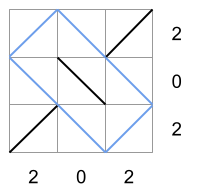## 채점 및 기타 정보

• 예제는 채점하지 않는다.